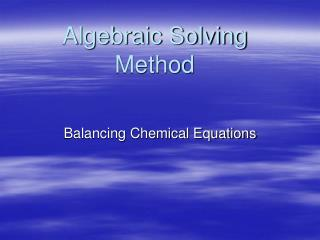DownloadDownload PresentationAlgebraic Solving Method

# Algebraic Solving Method

Télécharger la présentation## Algebraic Solving Method

- - - - - - - - - - - - - - - - - - - - - - - - - - - E N D - - - - - - - - - - - - - - - - - - - - - - - - - - -
##### Presentation Transcript

1. Algebraic Solving Method Balancing Chemical Equations

2. Balance This Equation Pb(N3)2 + Cr(MnO4)2  Cr2O3+ MnO2 + Pb3O4 + NO

3. Pb(N3)2 + Cr(MnO4)2  Cr2O3+ MnO2 + Pb3O4 + NO Let Pb(N3)2 = A Let Cr(MnO4)2 = B Let Cr2O3= C Let MnO2= D Let Pb3O4= E Let NO= F Give Each Compound a Variable

4. Solve For Each of the Variables Pb(N3)2+Cr(MnO4)2Cr2O3+MnO2+Pb3O4+NO Let A=1 • Pb: A=3E * A is the amount of Pb on the left side of the equation *3E is the amount on the left side

5. A=1 Pb: A=3E N: 6A=F Cr: B=2C Mn: 2B=D O: 8B=3C+2D+4E+F Substitute in A Pb: 1=3E  1/3=E N: 6x1=F  6=F Cr: B=2C or B/2=C Mn: 2B=D or B=D/2 O: 8B=3C+2D+4E+F 8B=3(B/2)+3(2B)+4(1/3)+6 Solve For Each of the Variables Pb(N3)2+Cr(MnO4)2Cr2O3+MnO2+Pb3O4+NO

6. Solve for Oxygen O: 8B=3B/2+4B+4/3+6 Find a lowest common multiple of 2 and 3 Multiply each side by 6 [6x] 8B=(3B/2)+(4B+4/3)+6 48B=9B+24B+8+36 48B=33B+44 15B=44

7. A=1 B=44/15 C=(B/2)x(44/15) C=44/30 D=2B D=(2/1)x(44/15) D=88/15 E=1/3 F=6 Find a GCD and multiply each. A=15 B=44 C=22 D=88 E=5 F=90 Simplify Your Fractions

8. Now You Have A Finished Equation Substitute each value into the equation. A=15 D=88 B=44 E=5 C=22 F=90 15Pb(N3)2 +44 Cr(MnO4)2  22Cr2O3+ 88MnO2 + 5Pb3O4 +90 NO

9. This powerpoint was kindly donated to www.worldofteaching.com http://www.worldofteaching.com is home to over a thousand powerpoints submitted by teachers. This is a completely free site and requires no registration. Please visit and I hope it will help in your teaching.